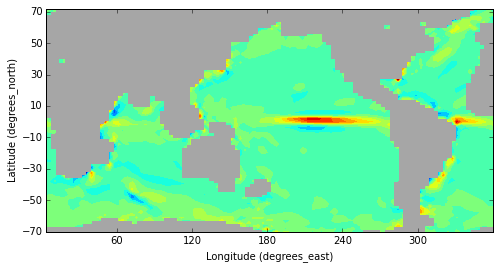# Spacegrids Documentation¶

Spacegrids is an open source library providing a Numpy array with grids, labelled axes and associated grid-related methods such as regridding and integration. Spacegrids provides an object data model of Netcdf data that ensures consistency between a Numpy data array and its grid under common operations (and so avoiding common pitfalls related to axis interpretation), and much more. It is a write less do more library for everyday use. These Interactive Netcdf data plots using d3.js are based on Spacegrids.

The Spacegrids module is a bit like "Numpy on grids". It allows code like:

TEMP = P['some_dataset']['temperature']
V = P['some_dataset']['y_velocity']
TV = TEMP*V
zonal_mean_TV = TV.mean(X)
TEMP2 = P['some_other_dataset']['temperature'] # defined on a different grid
deltaTEMP = TEMP - TEMP2.regrid(TEMP.grid)



It allows easy manipulation of spatial data, for instance a spatial grid of discrete latitude-longitude-depth coordinates covering the Earth's surface. This type of data is often stored in Netcdf file format, and indexed according to axes that are fully described in the Netcdf metadata. This allows interpretation of the data, consisting of the construction of axis and grid objects to be linked with a Field. This contains the data array (e.g. temperature) when loading data into memory. Axis alignment upon multiplication and other operations with fields is then automatic, and no knowledge is required of the data index order. The module maintains a focus on notational simplicity, ease of use and maintenance of consistency between data and grid information under data manipulation. New coordinate objects and grids can be naturally constructed from experiment parameters or aggregated data values.

## Features¶

• A numpy array with grid allowing automatic alignment and dimension broadcasting
• Easy to use and intuitive regridding functionality
• A data object model corresponding closely to Netcdf
• Easier IO via abstraction of IO with multiple Netcdf files
• Makes working with output of many experiments easy via aggregation methods
• The Field class eliminates errors arising from picking the wrong array index
• Quicker plotting due to automatic labels, axes etc.
• Distance-related methods such as spatial differentiation and integration on sphere
• Extensive unit tests and documentation

There is lots of documentation, both in the source code and elsewhere. Other documentation can be found at:

## Installation¶

Install spacegrids simply by running (on command line):

pip install spacegrids



On Mac, pip can be installed via "sudo easy_install pip". On Ubuntu/ Debian, install dependencies via package manager if pip install fails:

apt-get install python-{tk,numpy,matplotlib,scipy}



## License¶

The project is licensed under the BSD license.

## Tutorial¶

In this tutorial, we will analyse some real output from the UVic climate model. We will compare ocean temperatures and circulation between the imaginary case where the Drake Passage (between Antarctica and South America) is closed, and one where it is open (as it is today). I am interested to hear feedback, and you are welcome to e-mail me.

# Data files and initializing a project¶

### Data on disk¶

Before using the spacegrids module for data analysis, some minimal organizing of your Netcdf data files inside directories is helpful. The module looks for projects inside the ~/PROJECTS directory tree. Create ~/PROJECTS and mark a subdirectory of ~/PROJECTS, say test_project as a project directory simply by including a text file named projname containing the name of the project (e.g. echo foo > projname for a project named foo). Passing the nonick = True argument to sg.info and sg.Project removes the need for the projname file (see below). This is recommended for initial use. Subdirectories, and Netcdf files placed alongside them, in such a project directory (test_project) are interpreted as experiment output. So both a directory and a Netcdf file can be associated with an experiment. The case where a subdirectory represents an experiment allows the added advantage of being able to store the configuration files that were used by the numerical (Fortran or C) experiments alongside their Netcdf output files. There is a natural framework in spacegrids for parsing these configuration files and adding the parameters to exper objects, allowing the subsequent creation of new coord and axis objects across experiments from these parameters (e.g. CO2 concentrations, specified in the configuration file, might vary across experiments: maybe you would like to see how the average air temp and other quantities depend on it, casting pCO2 as a Coord). However, basic productive use of spacegrids is much simpler than this.

We will analyze some climate model data using a ~/PROJECTS tree containing two numerical model output files (experiments) and one file containing observational data (also captured in an experiment object), in this case each placed within their own experiment directory. This example project tree can be obtained by: wget http://web.science.unsw.edu.au/~wsijp/code/examples.tar.gz. (Unpacking is done inside the \$HOME directory using tar xvzf examples.tar.gz, unless a different root path is set.) This provides a project called test_project (note the text file called projname). The UVic climate model output consists of equilibrium fields where the model has been run with two geographic configurations. One where the Drake Passage has been closed by introducing an artificial land bridge (the DPC subdirectory), and one where the passage remains open (the DPO subdirectory). In addition, there is some observational data, the file Lev.cdf placed alongside the two experiment directories, to compare the model results to.

### Getting started¶

With the directory tree in place, we can start analyzing the data.

In :
import spacegrids as sg

In :
import numpy as np
import matplotlib.pyplot as plt
figsize(8, 4)


### Overview of the available data¶

The info function returns a dictionary of all the available project names and their paths. Here, we have one project (named my_project). Make sure there is at least one project listed when following these examples.

In :
D = sg.info_dict()

In :
D

Out:
{'my_project': '/home/wim/PROJECTS/test_project/'}

Using ls to examine the project directory content for test_project, we see two experiment directories and one Netcdf file. The sg module will interpret all three as experiment objects.

In :
ls /home/wim/PROJECTS/test_project/

DPC/  DPO/  Lev.cdf*  projname


To obtain a summary of the available projects while obtaining the paths, use D = sg.info(). To start a project instance, named P, it is easy to use:

In :
P = sg.Project(D['my_project'])

Creating exp object DPO from /home/wim/PROJECTS/test_project/
Creating exp object DPC from /home/wim/PROJECTS/test_project/
Creating exp object Lev.cdf from /home/wim/PROJECTS/test_project/
OK. Inferring direction from dimension name X itself. Renaming Coord to X_crd.
OK. Inferring direction from dimension name Z itself. Renaming Coord to Z_crd.
OK. Inferring direction from dimension name Y itself. Renaming Coord to Y_crd.

 spacegrids/fieldcls.py:4507: UserWarning:(mild): no units assigned to pft in cdfsniff
spacegrids/fieldcls.py:4507: UserWarning:(mild): no units assigned to type in cdfsniff
spacegrids/fieldcls.py:4507: UserWarning:(mild): no units assigned to pft_edges in cdfsniff
spacegrids/fieldcls.py:4507: UserWarning:(mild): no units assigned to type_edges in cdfsniff


Ignore the harmless warnings. They relate to the interpretation of the axes and dimension names found in the Netcdf files. Note that the Lev.cdf experiment object is named after the file, whereas the other two are named after the directories. Passing the nonick = True option would have been: D = sg.info(nonick=True) and P = sg.Project(D['my_project'],nonick = True). In this case, no projname file would be required, and the project would receive the same name as the directory, unless the name is specified. Upon project initialization, meta data associated with the grids, dimensions and axis directions is collected from the data files and interpreted. The project is now initialized. Subdirectories of the test_project directory correspond to (numerical) experiments and also Exper objects. If Netcdf files had been present in the test_project directory, they would have been interpreted as experiments. In this case, an Exper object (experiments) corresponds not to subdirectory, but a single Netcdf file. This latter arrangement corresponds to the structure of a project saved with P.write(), where all experiments are written to experiment files. If a path to a file (not project directory) is provided to sg.Project, a project is initialised assuming all project is contained in that single file.

# The Field class¶

### Fields and their grids¶

To look at an example of fields (e.g. temperature or humidity), it is best to load one into the project.

In :
P.load('O_temp')

OK. Fetched Field O_temp for DPO. 1 file found.
OK. Fetched Field O_temp for DPC. 1 file found.
O_temp for Lev.cdf could not be read.


The ocean temperature Field is now loaded into memory and associated with the project P. How do you know what variable name to pick? Inspection on the Bash command line of the Netcdf file with ncdump -h usually provides this information. Alternatively, experiments have an ls function: P['DPO'].ls() would yield a long list of available variables to be loaded as fields.

Notice that O_temp has not been loaded for Lev. This is because the Netcdf file inside the Lev directory follows different naming conventions. Inspection of the Netcdf file in the Lev directory reveals that temperature is referred to as otemp. To load it, we use:

In :
P.load('theta')

 spacegrids/fieldcls.py:4689: RuntimeWarning:invalid value encountered in equal
spacegrids/fieldcls.py:4689: RuntimeWarning:invalid value encountered in isnan

theta for DPO could not be read.
theta for DPC could not be read.
OK. Fetched Field theta for Lev.cdf. 1 file found.

 spacegrids/fieldcls.py:4708: RuntimeWarning:invalid value encountered in equal


Now all three temperature fields are loaded. We access the temperature fields of the DPO experiment and the observations Lev and test resulting field instances:

In :
TEMP = P['DPO']['O_temp']
TEMP2 = P['Lev.cdf']['theta']

TEMP # a field

Out:
O_temp

The shape of a Field corresponds to the shape of the Numpy array it contains and is consistent with the shape of its grid:

In :
TEMP.shape # show the shape of the numpy array containing the data. This is a 3D field

Out:
(19, 100, 100)
In :
TEMP.grid # show the grid on which the temp data is defined

Out:
(depth, latitude, longitude)

The names of the grid components depth, latitude and longitude correspond to the names used in the Netcdf file. For instance, there are different naming conventions for the Lev data:

In :
TEMP2.grid

Out:
(Z_crd, Y_crd, X_crd)

In fact, the Coord (dim) names in the Lev.cdf file were X, Y, Z, but since these names coincide with the axes names, they have been automatically renamed with the _crd suffix to distinguish them from the axes:

In :
TEMP2.grid.axis

Out:
Z

Fields can be saved to Netcdf again, along with all their metadata and coordinates via their write method, e.g. TEMP2.write(). To specify the path for instance: TEMP2.write(path = '/home/me/'). Experiment and coord objects also have save methods. In the case of an experiment, all loaded fields are saved to one file. An entire project can be saved with P.save(), yielding a project file structure (directory containing a projname text file and Netcdf files corresponding to experiments). Generally, it is good to provide a name argument different from the project name, and safeguards exist to avoid overwriting the original project on disk, but no safeguards exist yet to check for multiple instances of the same project name (defined in the projname file): for now, this is up to the user to check. Finally, an arbitrary list of fields can be saved with sg.nugget.

An overview of the experiments and loaded fields corresponding to the project are shown by:

In :
P.show() # the registered experiments can be viewed here

---- my_project ----
DPC         	DPO         	Lev.cdf

Project using 9.62 Mb.


And for an experiment:

In :
P['DPO'].show() # the loaded fields can be viewed here

DPO
----------
O_temp

Exper using 0.73 Mb. 1 field loaded.


### Slicing, plotting and interpolation¶

Objects representing general coordinate directions (X,Y axes etc) have been constructed during initialization of project P, and can be accessed as follows:

In :
P['DPO'].axes

Out:
[T, X, Y, Z]

Bring these axes into the namespace under their own names:

In :
for c in P['DPO'].axes:
exec c.name + ' = c'


So that we can reference them:

In :
X

Out:
X

Field objects allow slicing by reference to axis name and using ndarray index values. Here, we use the axis object, followed by a slice object (e.g. TEMP[X,5] or TEMP[Z,:] or TEMP[Y,55:] or TEMP[X,slice(55,None,None))]. We will refer to the use of Ax and Coord objects in __getitem__ as "hybrid slice notation". To plot the surface temperature using sg version of contourf:

In :
sg.contourf(sg.squeeze(TEMP[Z,0]))
clb = plt.colorbar()
clb.set_label(TEMP.units)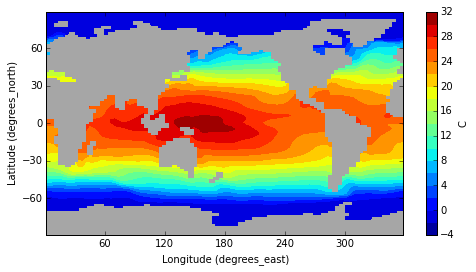The sg module automatically selects sensible contour levels and x,y ticks. Versions older than 1.1.4 lack this functionality. The number of levels can be set using argument num_cont. Alternatively, the Matplotlib levels arguments can be passed. Labels and orientation are extracted from the grid metadata. The field grid is used to extract plotting information, unless another grid is specified in the grid argument.

In :
sg.contourf(sg.squeeze(TEMP[X,50])) # a section at index 50.
clb = plt.colorbar()
clb.set_label(TEMP.units)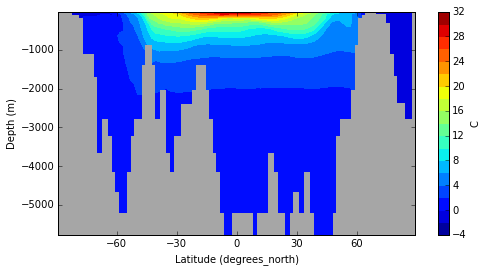Slicing can also be done via indices using the normal ndarray notation:

In :
(TEMP[0,:,:]).shape == (TEMP[Z,0]).shape

Out:
True

Another note of warning on stale objects. Slicing using Ax objects is a common case where stale objects after a module reload lead to strange errors. If we were to reload the module in the above case, and bring the X,Y,Z,T Ax objects into the namespace again, but use the now stale old TEMP field, the above slicing with Z will lead to a perplexing error. All objects will need to be refreshed in this case, which means redoing all the previous steps. In most common cases a module reload is not required, and this type of confusion arises mostly in module code development situations.

The load method (of the Project and Exper classes) also allows memory loading of subsets of the data only by passing the slices argument:

In :
P.load('O_sal',slices=(Z,0,X,50))

OK. Fetched Field O_sal for DPO. 1 file found.
OK. Fetched Field O_sal for DPC. 1 file found.
O_sal for Lev.cdf could not be read.

In :
P['DPO'].show()

DPO
----------
O_temp                         O_sal_sliced

Exper using 0.73 Mb. 2 fields loaded.

In :
P['DPO']['O_sal_sliced'].grid

Out:
(latitude)

Means are calculated easily by division.

In :
# Determine zonal mean mT of TEMP.

mTEMP = TEMP/X
mTEMP2 = TEMP2/X

 spacegrids/fieldcls.py:3342: RuntimeWarning:invalid value encountered in multiply
spacegrids/fieldcls.py:3505: RuntimeWarning:invalid value encountered in isnan


Basin-wide means are calculated using the maskout method to mask out all values outside the domain enclosing the node (a x,y location) argument. maskout uses a floodfill algorithm to mask the basin up to its boundaries. Note the hybrid notation for the node coordinates.

In :
# mask out the world ocean outside the Atlantic with NaN and take zonal mean.

mTEMP_masked=(TEMP[Y,33:].maskout(node = (X,85,Y,30) ) )/X # node is a point in the Atlantic


Plotting these two means for the upper 1600m or so:

In :
# --- start figure ---

lbl = ord('a')

pan = 0

height = 2
width = 1

rows = 2
cols = 1

ax = plt.subplot2grid((height, width), (int(np.floor(pan/cols)), pan%cols) )

# use the sg wrapper for the contour function.
cs= sg.contourf(mTEMP[Z,:10],linestyles='solid',showland=True); # slice only upper ocean
plt.tick_params(axis='x',labelbottom='off')
plt.xlabel('')
plt.ylabel('Depth (m)')
tle = plt.title('('+ chr(lbl) +') Global upper ocean temp ')

lbl += 1
pan += 1

ax = plt.subplot2grid((height, width), (int(np.floor(pan/cols)), pan%cols) )

cs= sg.contourf(mTEMP_masked[Z,:10],linestyles='solid',showland=True);  # slice only upper ocean

# Overiding the xlabel assigned by sg:
plt.xlabel('Latitude')
tle = plt.title('('+ chr(lbl) +') Atlantic upper ocean temp ')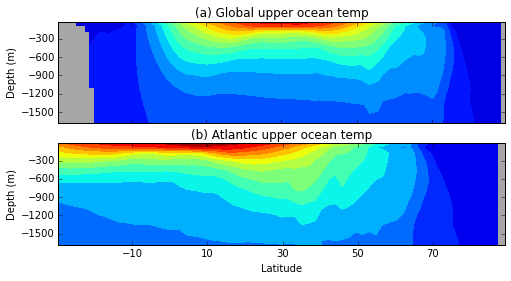In :
mTEMP.grid  # the dimensionality of the zonal mean is less

Out:
(depth, latitude)

A calculated field such as mTEMP can be inserted into the project using the insert method. This is generally not needed, but can be useful when the project or experiment is later written to disk. The insert method takes (name,value) pairs. If the value is a field, it is inserted into the vars attribute under name, otherwise into params.

In :
P['DPO'].insert( ('mtemp', mTEMP ) )


Now, mtemp is part of the DPO experiment:

In :
P['DPO'].show()

DPO
----------
O_temp                         O_sal_sliced
mtemp

Exper using 0.73 Mb. 3 fields loaded.


To see the effect of opening Drake Passage in the climate model:

In :
sg.contourf(sg.squeeze(P['DPO']['O_temp'][Z,0]-P['DPC']['O_temp'][Z,0]),cmap = mpl.cm.RdYlBu_r,levels = range(-8,9,1))
clb = plt.colorbar(ticks = range(-8,9,2))
clb.set_label(TEMP.units)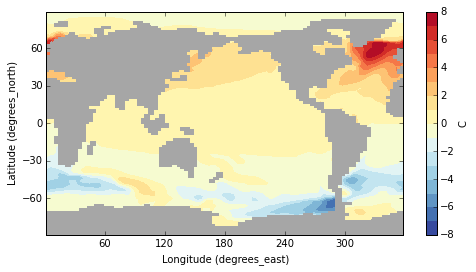Data is generally defined on different grids, so interpolation is needed. In our example, the observations are defined on a finer grid than the model data:

In :
TEMP2.shape

Out:
(33, 180, 360)

To overlay a plot of the model geography on atmospheric fields, we can load the ocean bathymetry field G_kmt, interpolate it on a finer grid with sg.finer_field (to avoid angled contours and show true resolution) and use contour plot over the 0.5 contour to capture the transition from land (value 0) to ocean(values 1 to 19). Here, we overlay this geographic outline over sea level air temperature:

In :
P.load(['G_kmt','A_slat'])
KMT = P['DPO']['G_kmt']
SAT = P['DPO']['A_slat']
nc = 10
sg.contourf(SAT, num_cont = nc,cmap = mpl.cm.RdYlBu_r)
cs=sg.contour(SAT,linestyles='solid', num_cont = nc, colors = 'k')
plt.clabel(cs,fmt='%1.0f')
sg.contour(sg.finer_field(KMT), colors='k',levels=[0.5,]  )

OK. Fetched Field G_kmt for DPO. 1 file found.
OK. Fetched Field G_kmt for DPC. 1 file found.
G_kmt for Lev.cdf could not be read.
OK. Fetched Field A_slat for DPO. 1 file found.
OK. Fetched Field A_slat for DPC. 1 file found.
A_slat for Lev.cdf could not be read.

 spacegrids/fieldcls.py:4711: UserWarning:missing value not set to NaN.

Out:
<matplotlib.contour.QuadContourSet instance at 0xaed210ac>
 /usr/local/lib/python2.7/dist-packages/matplotlib/text.py:52: UnicodeWarning:Unicode equal comparison failed to convert both arguments to Unicode - interpreting them as being unequal
/usr/local/lib/python2.7/dist-packages/matplotlib/text.py:54: UnicodeWarning:Unicode equal comparison failed to convert both arguments to Unicode - interpreting them as being unequal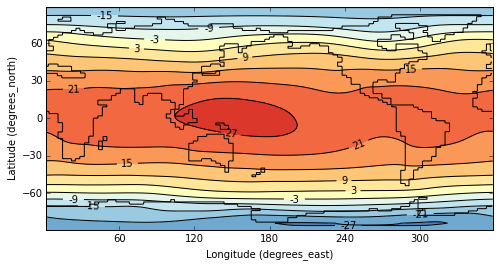To interpolate a field F in Spacegrids, simply call the regrid method of F on the desired grid gr as F(gr). For instance, to compute the difference between the zonal mean of the model and the observations:

In :
dmTEMP = mTEMP.regrid(mTEMP2.grid) - mTEMP2


As a shorthand, call the regrid method simply by calling the field itself on the target grid:

In :
dmTEMP = mTEMP(mTEMP2.grid) - mTEMP2

In :
cont_int = np.arange(-6.,6.,1.)
cmap = mpl.cm.RdYlBu_r	# Colormap red yellow blue reversed
pl1 = sg.contourf(dmTEMP,  levels = cont_int, cmap = cmap);

clb = plt.colorbar(ticks = cont_int)
clb.set_label(TEMP.units)
plt.xlim([-80.,70.])
plt.xticks([-60,-30,0,30,60])
plt.ylabel('Depth (m)')
tle = plt.title(' Zonal avg. T difference model - observations')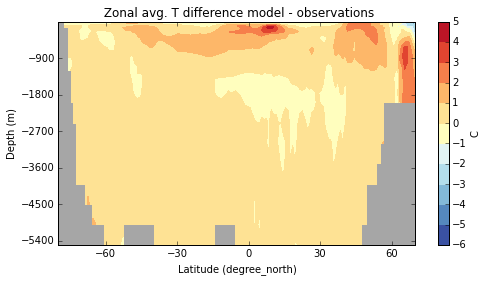The model is pretty good! It is generally a bit too warm (perhaps due to the use of post-industrialized CO2). The resulting grid is defined on that of Lev:

In :
dmTEMP.grid # the resulting grid is that of the Lev data

Out:
(Z_crd, Y_crd)

We have seen that it is easy to regrid data. This is also the case for data defined on grids of different dimensions. The ocean exhibits siginificant variations from its mean with longitude, leading to differences in local climate. How would we compare the sea surface temperature to its zonal mean? The following is bound to give an error, as the zonal mean is defined on a different (smaller dimensional) grid than temperature:

In :
sTEMP = TEMP[Z,0]# slice to obtain surface temperature
dTEMP = sTEMP - mTEMP[Z,0]

---------------------------------------------------------------------------
Exception                                 Traceback (most recent call last)
<ipython-input-38-1428ceee0c29> in <module>()
1 sTEMP = TEMP[Z,0]# slice to obtain surface temperature
----> 2 dTEMP = sTEMP - mTEMP[Z,0]

/home/wim/github/spacegrids/spacegrids/fieldcls.pyc in __sub__(self, other)
3132
3133       else:
-> 3134         raise Exception('Field shape error in %s-%s with Field %s: shapes must match. Try F - G(F.grid) or F(G.grid) - G.' % (self,other,self)  )
3135
3136

Exception: Field shape error in O_temp_sliced-O_temp_sliced with Field O_temp_sliced: shapes must match. Try F - G(F.grid) or F(G.grid) - G.

The error message provides a hint: regrid one term. regridding mTEMP[Z,0] onto sTEMP.gr expands the right term (by creating equal copies in the X direction, this is called broadcasting) to yield the right shape:

In :
dTEMP = sTEMP - (sg.squeeze(mTEMP[Z,0])).regrid(sTEMP.grid)

In :
pl1 = sg.contourf(sg.squeeze(dTEMP), cmap = mpl.cm.RdYlBu_r)
clb = plt.colorbar()
clb.set_label('C')
tle = plt.title('Sea Surface Temperature difference from zonal mean')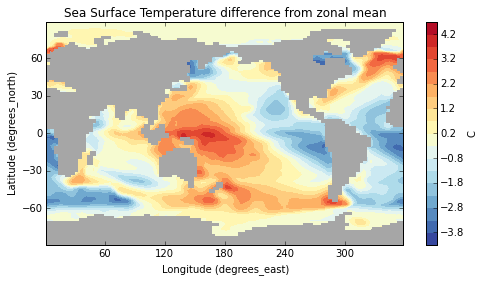The North Atlantic is warmer than at similar Pacific latitudes, leading to a milder western European climate. A pronounced zonal temperature difference is also apparent in the tropical Pacific (related to the "warm pool").

To compute the meridional streamfunction, we load the velocity in the y direction and apply the calculations in simple notation:

In :
# Give the experiment objects convenient names E, E2:
E = P['DPO'];E2 = P['DPC']
varname = 'O_velY'

# load velocity by netcdf name.
P.load(varname)

# obtain velocity as sg field object V from project.
V = E[varname]
V2 = E2[varname]

# take the meridional stream function by taking zonal grid-integral X*V
# and the vertical primitive of the result along Z using the pipe.
PSI = Z|(X*V)*1e-6
PSI2 = Z|(X*V2)*1e-6

OK. Fetched Field O_velY for DPO. 1 file found.
OK. Fetched Field O_velY for DPC. 1 file found.
O_velY for Lev.cdf could not be read.


Now that we have the required fields, we can plot them.

In :
lvls = np.arange(-100,100,4)
xlims = [-80,70]
ylims = [-4500., -0.]

# --- start figure ---

lbl = ord('a')

pan = 0

height = 2
width = 1

rows = 2
cols = 1

F = PSI
ax = plt.subplot2grid((height, width), (int(np.floor(pan/cols)), pan%cols) )

# use the sg wrapper for the contour function.
cs= sg.contour(F,linestyles='solid',showland=True,levels = lvls,colors='k');

plt.clabel(cs,fmt='%1.1f');

plt.xlim(xlims)
plt.ylim(ylims)
plt.tick_params(axis='x',labelbottom='off')
plt.xlabel('')
plt.ylabel('Depth (m)')
tle = plt.title('('+ chr(lbl) +') MOC Drake Passage open ')

lbl += 1
pan += 1

F = PSI2
ax = plt.subplot2grid((height, width), (int(np.floor(pan/cols)), pan%cols) )

cs= sg.contour(F,linestyles='solid',showland=True,levels = lvls,colors='k');
plt.clabel(cs,fmt='%1.1f');
plt.xlim(xlims)
plt.ylim(ylims)

# Overiding the xlabel assigned by sg:
plt.xlabel('Latitude')
tle = plt.title('('+ chr(lbl) +') MOC Drake Passage closed ')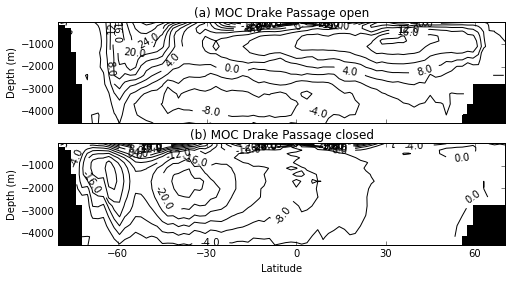There is strong southern sinking in the DP closed case, and a switch to northern sinking in the DP open case. That's why there was warming in the NH.

Again, the Atlantic MOC can be computed by using the maskout of Fields, using V_masked = V[Y,33:].maskout(node = (X,85,Y,30) ) instead of V (see above description for zonal temperature mean). See our special recipy for this.

Field concatenation is done with the concatenate function, similar to Numpy. If we split surface air temperature SAT latitudinally into three pieces:

In :
SAT1=SAT[Y,:40];SAT2=SAT[Y,40:50];SAT3=SAT[Y,50:]


And re-assemble along the default axis ax = None, sg will guess to use the incomplete (differing) axes:

In :
SAT_cat = sg.concatenate([SAT1,SAT2,SAT3])


(This yields the same result as sg.concatenate([SAT1,SAT2,SAT3],ax=Y).) Drawing the zonal mean shows that SAT has been re-assembled correctly:

In :
(SAT_cat/X).draw()

Out:
([<matplotlib.lines.Line2D at 0xaea1806c>], None)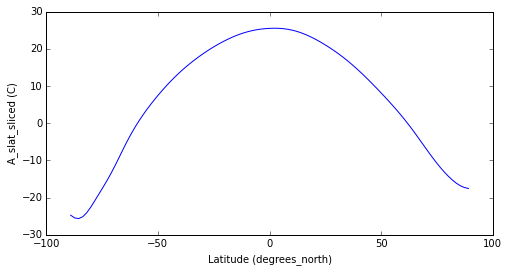If multiple files are found in an experiment directory, and they contain the same field, sg will assume they are pieces of the same field and concatenate them upon loading. An example would be files containing different time slices of the same field (e.g. temperature).

Tip: field objects have a squeeze and unsqueeze method: use help to learn more about them. Fields are squeezed upon loading and unsqueezed when saved.

### Grids and coord objects¶

Grid (Gr) objects model the grids on which the field data is defined. They consist of tuples containing Coord objects. Coord objects consist of points along an axis and are read and named from the Netcdf files. To bring these objects into the namespace under their own name:

In :
for c in E.cstack:
exec c.name +' = c'


This allows us to reference the Coord objects built from the Netcdf file by the names extracted from the metadata:

In :
depth[:] # depth coordinate points

Out:
array([   17.5,    82.5,   177.5,   302.5,   457.5,   642.5,   857.5,
1102.5,  1377.5,  1682.5,  2017.5,  2382.5,  2777.5,  3202.5,
3657.5,  4142.5,  4657.5,  5202.5,  5777.5])
In :
latitude[:10] # latitudinal coordinate points (truncated)

Out:
array([-89.09999847, -87.30000305, -85.5       , -83.69999695,
-81.90000153, -80.09999847, -78.30000305, -76.5       ,
-74.69999695, -72.90000153])

The result of the __getitem__ method here is the ndarray containing the data of the coordinate points. It is important to realize that these coord names (depth, latitude etc) have been read from the Netcdf file and have not been hard coded into Spacegrids.

A shorthand for grid construction from Coord objects is via multiplication:

In :
latitude*longitude

Out:
(latitude, longitude)
In :
latitude*longitude*depth

Out:
(latitude, longitude, depth)

The latter 3 dimensional grid is associated with ndarray data where the first index represents latitude, the second longitude and the third depth. Field objects contain a grid object that is always congruent with the ndarray holding the field data. The grid objects contained in fields are used to always ensure data alignment, for instance under multiplication.

Coord objects and grid objects are not the same. To construct a one dimensional Gr (grid) object:

In :
latitude**2

Out:
(latitude)

### Axis alignment¶

We had:

In :
TEMP.grid

Out:
(depth, latitude, longitude)

With data in the ndarray:

In :
(TEMP[:]).shape

Out:
(19, 100, 100)

where we recognise the length of the depth coord as 19:

In :
len(depth[:])

Out:
19

Rearrangement of the coordinates:

In :
TEMP_shuffled = TEMP(latitude*longitude*depth)
TEMP_shuffled.grid

Out:
(latitude, longitude, depth)
In :
(TEMP_shuffled[:]).shape

Out:
(100, 100, 19)

Data axis alignment is automatic. For instance, multiplying temperature with velocity:

In :
F = TEMP*V

In :
F.grid

Out:
(depth, latitude, longitude)

Multiplication alignment works regardless of the order of the coordinates:

In :
F2 = TEMP_shuffled*V
F2.grid

Out:
(latitude, longitude, depth)

Here, V is actually defined on a different grid: the velocity grid:

In :
V.grid

Out:
(depth, latitude_V, longitude_V)

Why then is the result of TEMP*V defined on the temperature grid? This is because multiplication automatically triggers interpolation of the right multiplicant to the grid of the left multiplicant. Vice versa:

In :
(V*TEMP).grid

Out:
(depth, latitude_V, longitude_V)

### Axis objects¶

The objects P['DPO'], P['DPC'] and P['Lev'] are experiment objects, and correspond to the directories containing the Netcdf files. Different data ("experiment") files may contain data defined on different grid and use different coordinate naming conventions. A list of the coord objects associated with an experiment object is accessed from the .cstack attribute:

In :
P['Lev.cdf'].cstack

Out:
[X_crd, Z_crd, Y_crd]
In :
P['DPO'].cstack[:4] # truncated list of coord objects

Out:
[time, longitude, longitude_edges, latitude]

The depth coordinates differ for the model and the observational data.

However, both coord objects point in the same direction: Z. The name ("Z") of this direction has also been read from the Netcdf files. This is encapsulated in the ax class (axis).

In :
depth.axis

Out:
Z

Multiplication of a Gr (grid) with an Ax object (representing an axis) picks the Coord component along that axis.

In :
X*(latitude*longitude)

Out:
longitude

We also saw the Ax object being used to calculate zonal means. In the following example, we load the surface heat flux, demonstrate some operations using Ax objects and compute the poleward heat transport.

In :
# load heat flux by netcdf name.
P.load('F_heat')

# obtain oceanic heat flux as sg field object HF from project.
HF = P['DPO']['F_heat']
HF2 = P['DPC']['F_heat']

OK. Fetched Field F_heat for DPO. 1 file found.
OK. Fetched Field F_heat for DPC. 1 file found.
F_heat for Lev.cdf could not be read.


Again, ignore the harmless warning: the Lev data contains no heat flux data. The heat flux data is 2 dimensional, as can be seen from the grid:

In :
HF.grid

Out:
(latitude, longitude)

And looks like this:

In :
pl = sg.contourf(HF,levels = range(-200,200,20),cmap = mpl.cm.RdYlBu)
clb = plt.colorbar()
clb.set_label(HF.units)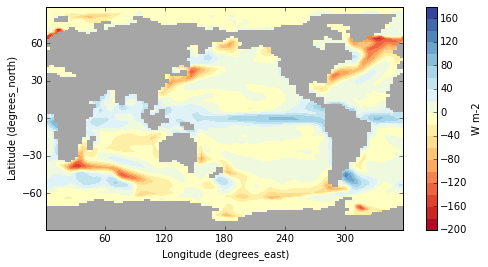Shift fields by reference to the Ax objects:

In :
HF_shifted = sg.roll(HF,coord = X,shift = 50)

In :
pl = sg.contourf(HF_shifted,levels = range(-200,200,20),cmap = mpl.cm.RdYlBu)
clb = plt.colorbar()
clb.set_label(HF.units)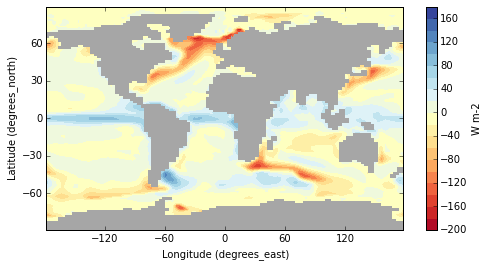In :
HF_shifted.grid

Out:
(latitude, longitude_rolled)

Note that the grid is updated along with the data (the zero meridian still passes through Greenwich), resulting in a different grid (longitude_rolled appears in place of longitude). This behaviour can be disabled.

Compute the zonal integral (using multiplication notation HF*X) followed by the primitive in the Y direction (using the pipe | notation):

In :
# Determine the total oceanic poleward heat transport via the y-primitive and X-intergral, and scale to Petawatt.

PHT = Y|(HF*X)*1e-15
PHT2 = Y|(HF2*X)*1e-15

In :
pl1, = sg.plot(PHT,color = 'b');
pl2, = sg.plot(PHT2,color='r');

plt.grid()
plt.xlim([-80.,80.])
plt.xticks([-60,-30,0,30,60])
plt.ylabel('PW')
tle = plt.title(' Ocean PHT (PW) ')
lgnd = plt.legend([pl1,pl2],['DP open','DP closed'],loc=2)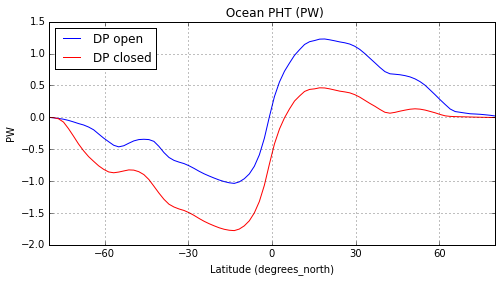Interesting, the Drake Passage closed configuration has more southward poleward heat transport. We could have used the coord names to compute the PHT:

In :
PHT = latitude|(HF*longitude)*1e-15


The axis names X,Y,... are a shorthand for these coord names, and are easier to use.

### More grid operations¶

Operations such as derivatives and integration are methods of the coord class:

In :
latitude.der # the derivative on the sphere in the latitudinal direction

Out:
<bound method YCoord.der of latitude>
In :
X.der

Out:
<bound method Ax.der of X>
In :
HF_shifted2 = longitude.coord_shift(HF,50)

In :
HF_shifted2.grid

Out:
(latitude, longitude_rolled)

Do we get the same result from both shifts? This is a shorthand to access the X coord of HF_shifted2:

In :
# obtain coord in X direction
X*(HF_shifted2.grid)

Out:
longitude_rolled

The two shifted coord objects are different objects

In :
# compare the ndarray content of the X components of the two shifted grids
X*(HF_shifted2.grid) == X*(HF_shifted.grid)

Out:
False

But contain the same values:

In :
((X*(HF_shifted2.grid))[:] == (X*(HF_shifted.grid))[:]).all()

Out:
True

Other derived Coord objects are obtained from slicing:

In :
HF_sliced = HF_shifted[Y,:36]

In :
pl = sg.contourf(HF_sliced,levels = range(-200,200,20),cmap = mpl.cm.RdYlBu)
clb = plt.colorbar()
clb.set_label(HF.units)
tle = plt.title('Heat flux in Southern Ocean')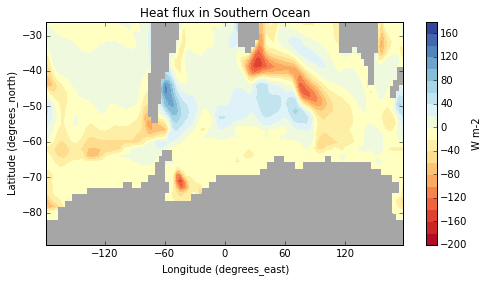Similar to the roll function, slicing gives a new grid:

In :
HF3 = HF[X,:50,Y,:36]

In :
HF3.grid

Out:
(latitude_sliced, longitude_sliced)
In :
len(Y*HF3.grid)

Out:
36

The sum method of a Coord object only sums the ndarray content of a Field:

In :
sTEMP = longitude.sum(TEMP)
sTEMP.grid

Out:
(depth, latitude)

Similar to Pandas, the NaN (np.nan) values are not counted in these operations. Similarly, the vsum method is the sum weighted with the volumes (lengths, areas) of the grid cells:

In :
help(longitude.vsum)

Help on method vsum in module spacegrids.fieldcls:

vsum(self, F) method of spacegrids.fieldcls.XCoord instance
Sums Field along self Coord, weighted with grid cell width (using self.d(), called by self.vol(F.grid)). Note: due to possible dependence of one Coord on the other, only use mean method of grid. There is no mean method for Coord objects.

Method of Coord  that sums Field F along self Coord direction, weighted with the grid cell width. Calls sum method. See sum method.

Calculation is self.sum(F*(self.vol(F.grid))). For grids with grid cell width depending on coordinates, use corresponding Gr methods.

Args:
F: (Field) field of certain dimension n to sum

Returns:
Field of dimension n-1 or float if n=1.

Raises:
ValueError: when Coord (self) is not in F.grid (grid of Field).



Similar for cumsum and vcumsum.

Grid objects also have volume weighted sum methods. These methods are preferred over their Coord counterparts as coordinate cell dimensions may depend on other coordinates (e.g. the distance between longitudinal arcs diminishes towards the poles), and grid objects take care of this.

In :
my_grid = latitude*longitude
my_grid.vsum(TEMP)[:]

Out:
array([  6.43187995e+15,   5.82743640e+15,   4.73322880e+15,
3.79210234e+15,   3.00009346e+15,   2.34159369e+15,
1.79678076e+15,   1.34723259e+15,   1.01153068e+15,
8.09927754e+14,   6.79646188e+14,   5.63901912e+14,
4.67317201e+14,   3.97696671e+14,   3.17287739e+14,
2.27232412e+14,   1.43312843e+14,   8.57740304e+13,
3.19512842e+13])
In :
my_grid.vsum(TEMP).grid

Out:
(depth)

The volume of the domain of non NaN values inside TEMP is found from:

In :
TEMP.grid.vsum(TEMP.ones())

Out:
1.3576934808828324e+18

This can also be achieved with the multiplication notation:

In :
TEMP.ones()*(X*Y*Z)

Out:
1.3576934808828324e+18

The ones method of Field yields a new Field, where all non- NaN values (of TEMP in this case) are set to 1 and the NaN values remain NaN. Here, the one values constitute the domain of ocean water, as NaN values indicate land (rock).

This allows a calculation of the mean ocean temperature in the model:

In :
TEMP.grid.vsum(TEMP)/TEMP.grid.vsum(TEMP.ones())

Out:
3.9464440090035096
In :
# more succinctly:
TEMP.grid.mean(TEMP)

Out:
3.9464440090035096

This can also be achieved with the division notation:

In :
TEMP/(X*Y*Z)

Out:
3.9464440090035096

The Ax class has a derivative method:

In :
# Calculate derivative in X direction:
dTEMPdX = X.der(TEMP)
dTEMPdX.grid

Out:
(depth, latitude, longitude)
In :
# compute the vertical temperature profile and plot it.
vpTEMP = TEMP/(Y*X)
pl, = sg.plot(vpTEMP)
lb = plt.xlabel('degrees C');tle = title('Vertical profile of average ocean temp')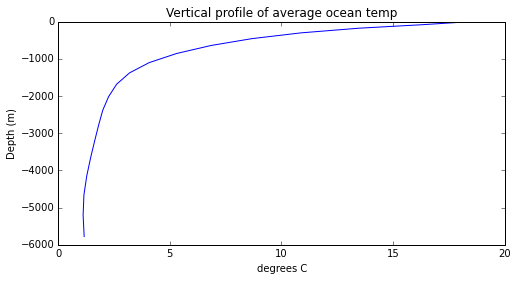In :
# take the vertical derivative of the vertical temperature profile and plot it.
pl, = sg.plot(Z.der(vpTEMP))
lb = plt.xlabel('degs C per m');tle = plt.title('Vertical temperature gradient')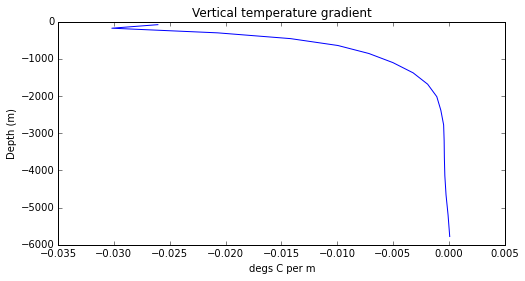Another notation, involving Ax objects, uses the ^ (carat) symbol:

In :
# test whether nparray content is the same. Excluding the first nan value.
((Z^vpTEMP).value[1:] == (Z.der(vpTEMP)).value[1:]).all()

Out:
True

The volume weighted cumulative sum method vcumsum of the Coord class, the primitive integral along that axis, is easily accessed with the pipe | notation and the ax class (here the instance Z). In the latter case the name of the particular coord need not be known.

In :
# test whether the depth coord method vcumsum yields the same result as Z|
(depth.vcumsum(vpTEMP)[:] == (Z|vpTEMP)[:]).all()

Out:
True

# Operators, divergences and vector fields.¶

Spacegrids allows representations of vector fields (e.g. velocity fields). We're loading the zonal velocity to examine a 2 dimensional vector fields constructed from U and V.

In :
# load the ocean velocity in the X direction.
P.load('O_velX')

OK. Fetched Field O_velX for DPO. 1 file found.
OK. Fetched Field O_velX for DPC. 1 file found.
O_velX for Lev.cdf could not be read.


Earlier, multiplication of two fields yielded a new field. In contrast, multiplying the two fields U and V does not result in a new field here. Instead, we get a container class holding the two fields. This container is the vfield class (vector field).

In :
# obtain horizontal velocity fields
U = P['DPC']['O_velX']
V = P['DPO']['O_velY']
# create a 2 dimensional vector field defined on 3 dimensional space
my_vector_field = U*V
my_vector_field

Out:
(O_velX, O_velY)

Why do these fields behave differently under multiplication? Fields have a direction attribute:

In :
U.direction

Out:
X
In :
V.direction

Out:
Y
In :
TEMP.direction

Out:
scalar

The velocity fields U and V have directions X and Y, obviously indicating the direction they are pointing in: they are "directional" fields. In contrast, temperature TEMP is marked as a scaler, this is a "scalar" field. In general, fields of like direction multiply to yield a new field containing the multiplied nparray data. For instance:

In :
# This gives a field
U*U

Out:
O_velX_times_O_velX

This gives a vector field of the velocity components squared:

In :
(U*V)*(U*V)

Out:
(O_velX_times_O_velX, O_velY_times_O_velY)

Vector fields have a direction method that yields a container of the directions of its component fields.

In :
my_vector_field.direction()

Out:
(X,Y,)

The sg module has its own wrapper to the quiver plot method of Matplotlib that takes vector fields.

The (field) Operator class and its derived classes (e.g. sg.Der, sg.Integ, sg.FieldMul etc.) allows the construction of operators that act on fields and vector fields. E.g. div and curl operators, etc. Ideas for useful operators are shown below. Operators, say K1 and K2, can be multiplied before they are applied. E.g. if K = K1*K2, no evaluation has occurred yet, and evaluation yields: K*F = K1(K2(F)). So K2 acts on F first, then K1 acts on the resulting field. the curl of a 2D vectorfield UV would then be curl(UV). IfUVis defined on 3D space, thecurl operator acts on each z-level. For instance, the X-derivative object can be instantiated as follows:

In :
# Instatiate an X-derivative operator object
ddX = sg.Der(X)
ddX

Out:
<spacegrids.ops.Der at 0xac4b276c>
In :
ddX*TEMP

Out:
O_temp

This is equivalent to calling the ddX field operator object on TEMP:

In :
ddX(TEMP)

Out:
O_temp

Applying field operators in succession:

In :
ddX*ddX

Out:
<spacegrids.ops.Mul at 0xac47e3ac>

Where:

In :
# Create second order X-derivative
d2dX2 = ddX*ddX
# Apply it to the temperature field
d2dX2*TEMP

Out:
O_temp

This is the same as successive calls of ddX on the Field:

In :
# Succesive calls of the ddX object
( (d2dX2*TEMP).value == ( ddX(ddX(TEMP)) ).value ).any()

Out:
True

We could have obtained the Z derivative of the vertical temperature profile above with an operator class:

In :
# Create Z-derivative object
ddZ = sg.Der(Z)
# Apply the derivative operator and plot the result
pl, = sg.plot(ddZ*vpTEMP)
lb = plt.xlabel('degs C per meter')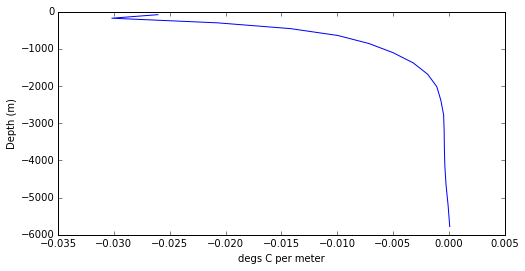Another useful field operator is Pick. It picks a component of a vector field:

In :
sg.Pick(X)*my_vector_field

Out:
O_velX

Here are definitions of some useful field operators:

In :
ddX = sg.Der(X) # derivative in X
ddY = sg.Der(Y) # etc
ddZ = sg.Der(Z)

pX = sg.Pick(X) # pick the component with direction X from vfield object (projection)
pY = sg.Pick(Y) # etc.
pZ = sg.Pick(Z)

mX = sg.Mean(X) # take zonal mean of any field argument or right-multiplicant.
mY = sg.Mean(Y) # etc
mZ = sg.Mean(Z)

sX = sg.Integ(X) # take integral
sY = sg.Integ(Y)
sZ = sg.Integ(Z)

intX = sg.Prim(X) # take primitive function of any field argument
intY = sg.Prim(Y)
intZ = sg.Prim(Z)

set2X = sg.SetDirection(X) # change the direction attribute of field argument to X
set2Y = sg.SetDirection(Y)
set2Z = sg.SetDirection(Z)
set2scalar = sg.SetDirection(sg.ID())

Avg = mZ*mX*mY  # a 3D average

curl = ddX*set2scalar*pY - ddY*set2scalar*pX      #  curl operator expressed in basic operators

div = ddX*set2scalar*pX + ddY*set2scalar*pY       # divergence

div3 = ddX*set2scalar*pX + ddY*set2scalar*pY + ddZ*set2scalar*pZ

grad = set2X*ddX + set2Y*ddY    # gradient. To be used on single field objects.

In :
# Take surface slices
sU = U[Z,0]
sV = V[Z,0]
surface_vectors = sU*sV
(div*surface_vectors).direction

Duplicate grids. FYI: replacing right gr.

Out:
scalar
In :
pl = sg.contourf(sg.squeeze((div*surface_vectors)[Y,10:-10]))